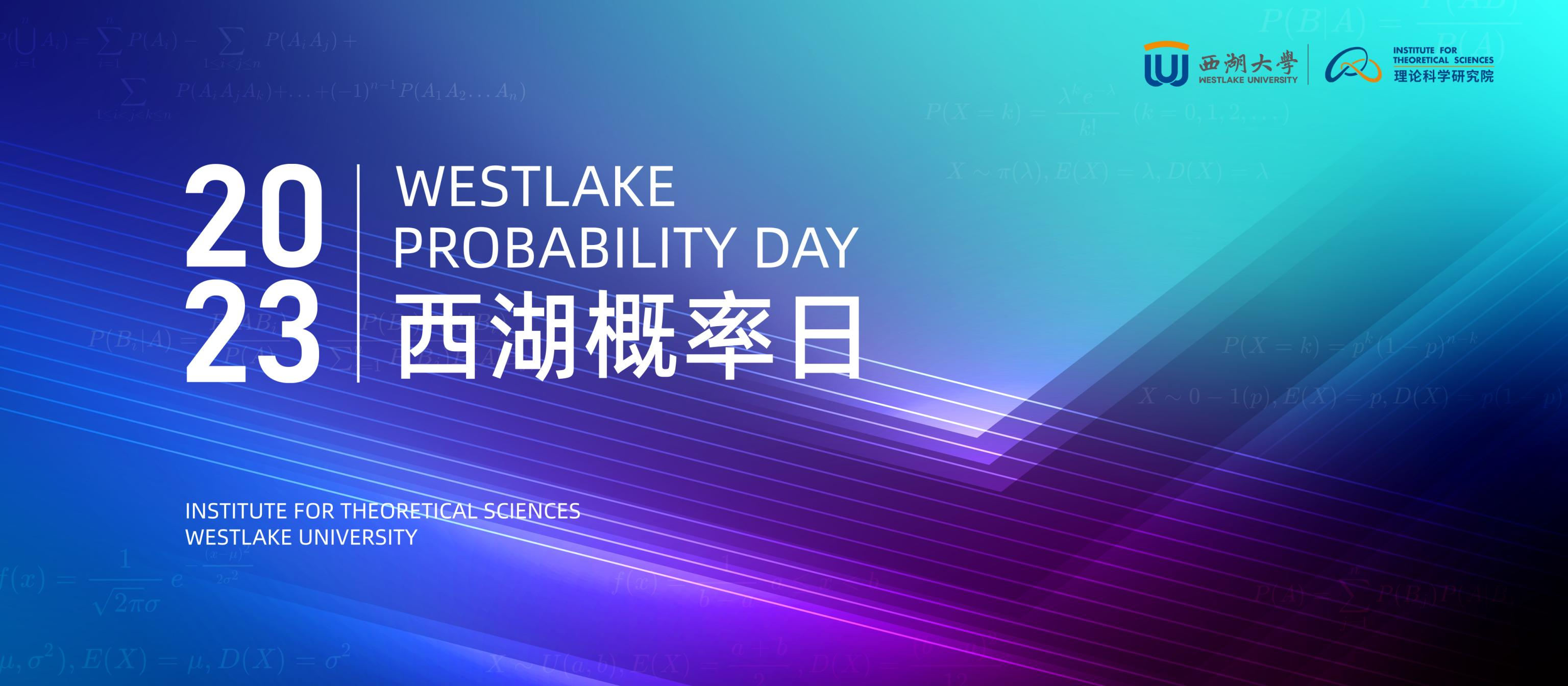﻿ 2023西湖概率日-西湖大学理论科学研究院# 2023西湖概率日

2023-03-31 08:04:429:30-10:30

Speaker: Hao Wu, Tsinghua University

Title: Connection probabilities for random-cluster model and uniform spanning tree

Abstract: Conformal invariance of critical lattice models in two-dimensional has been vigorously studied for decades. In this talk, we focus on connection probabilities of critical lattice models in polygons. This talk has two parts.

• In the first part, we consider critical random-cluster model with cluster weight $q\in (0,4)$ and give conjectural formulas for connection probabilities of multiple interfaces. The conjectural formulas are proved for q=2, i.e. the FK-Ising model.

• In the second part, we consider uniform spanning tree (UST) and give formulas for connection probabilities of multiple Peano curves. UST can be viewed as the limit of random-cluster model as $q$ goes to 0. Its connection probabilities turn out to be related to logarithmic CFT.

This talk is based on joint works with Yu Feng, Mingchang Liu, and Eveliina Peltola.

11:00-12:00

Speaker: Alejandro Ramírez, NYU Shanghai

Title: Balanced excited random walk in two dimensions

Abstract: We give non-trivial upper and lower bounds on the range of the so-called Balanced Excited Random Walk in two dimensions, and verify a conjecture of Benjamini, Kozma and Schapira. These are the first non-trivial results for this 2-dimensional model. This is a joint work with Omer Angel (University of British Columbia) and Mark Holmes (University of Melbourne).

14:00-15:00

Speaker: Elie Aidekon, Fudan University

Title: 1-d Brownian loop soup, Fleming--Viot processes and Bass--Burdzy flow

Abstract: We describe the connection between these three objects which appear in the problem of conditioning the so-called perturbed reflecting Brownian motion on its occupation field.

Joint work with Yueyun Hu and Zhan Shi.

15:30-16:30

Speaker: Dong Yao, Jiangsu Normal University

Title: Mean Field Behavior during the Big Bang regime for Coalescing Random Walks

Abstract: The talk is concerned with the coalescing random walk model on general graphs G. Initially every vertex of G has a particle. Each particle performs independent random walks. Whenever two particles meet, they merge into one particle which continues to perform a random walk. We set up a unified framework to study the leading order of the decay rate of P(t), which is the expectation of the fraction of occupied sites at time t, particularly for the ‘Big Bang’ regime where the time t<< T(coal):=E[inf{s: There is only one particle left at time s}].

Our results show that P(t) satisfies certain `mean field behavior', if the graphs satisfy certain ‘transience-like’ conditions. We apply this framework to two families of graphs: (1) graphs generated by configuration model with degree at least 3, and (2) finite and infinite vertex-transitive graphs. In the first case, (tP(t))^{-1} is approximately the probability that two particles starting from the root of the corresponding unimodular Galton-Watson tree never collide after one of them leaves the root. In the second case we establish similar results for finite ‘uniformly transient’ graphs and infinite transient transitive unimodular graphs. Based on joint work with Jonathan Hermon, Shuangping Li and Lingfu Zhang.